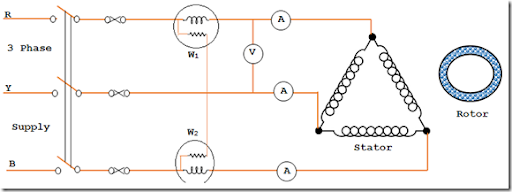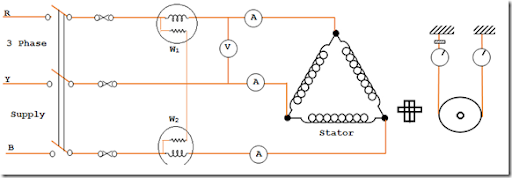# No Load Test and Locked Rotor Test in an Induction Motor

The various constant of the equivalent circuit of the induction motor can be determined as detailed below:

### Determination of Go and Bo and hence Ro and Xo:

To find out Go and Bo the induction motor is made to run at synchronous speed by using another machine. The additional machine helps to supply the friction and Windage losses, under this conditions, the slip s=0 and the current drawn by the induction motor is Io only since s=0, the term RL=R2{(1/s)-1)} becomes the load resistance RL=0. In this test the wattmeter reading, say

W=3GoV2,
where,
V is the supply voltage.
Therefore, Go = W/3V2
Also, No load current I0 = V.Yo
Therefore Yo=Io/V
Bo = √ (Yo2 – Go2)

Here, Go is the exciting conductor and Bo is the exciting susceptance. Knowing these values Ro and Xo can be determined. These quantities are required for drawing the equivalent circuit.
Normally it is not possible to run the induction motor at synchronous speed. If it coupled with another motor and whose speed can be varied up to the synchronous speed of the induction motor to run without any mechanical load on it. The speed is assumed to be synchronous speed. With this assumption the Go and Bo can determined as detailed below.The connection diagram for no load test on three phase induction motor is shown above. The wattmeter, ammeter and voltmeter, readings are taken. The total power input is given by the two wattmeters W1 and W2.

Let the total input power = W0 watts
No load input current = I0 amps
Voltage applied = Vo volts

At no load, the input power is supplied to meet out the losses.

The various losses are,
1. Stator winding loss 3Io2R1
2. Core loss 3GoV2
3. Friction and Windage losses.
The core loss, friction Windage losses totally are called fixed losses.
Since the total power input is known, and is equal to Wo and
Wo = √3VL I0 CosΦ0
From this relation
CosΦo = W / √3VLI0
Where VL = Line Voltage
I0 = Input current at no load
W0 = Input power at no load
From this test, Io, Wo, CosΦo are observed and determined.

### Locked Rotor TestThis test is also called as Locked Rotor test or short circuit test. Connection diagram of blocked rotor test on three phase induction motor is shown in the figure.

Under this test, the rotor is locked (not allowed to rotate or allowed to rotate at very slow speed). In case of slip ring induction motor, the rotor windings are short circuited at the slip rings. A reduced voltage, nearly 15% of normal voltage is applied to the stator winding. The voltage is so adjusted to allow full load current to the stator windings.

The power input, the current voltage applied is measured using the meters connected in the circuit. They are, Vs the short circuit voltage, IS, short circuit current with the voltage Vs, and Ws total power taken by the motor on short circuit.
From the measured values the followings are calculated:

#### 1. Short circuit current with respect to the normal supply voltage of the stator.

That is ISN, = Is x (V/Vs)
Where
ISN = Short circuit current w.r.t normal voltage
Vs = Reduced voltage applied during the short circuit
Is = Short circuit current with voltage applied during short circuit
V = Normal supply voltage to stator winding

#### 2. Power factor blocked rotor test

It is determined as, Ws = √3VsIsCosΦs
Therefore CosΦs = Ws / √3VsIs

Where Ws = Total power drawn by the motor on short circuit
V = Voltage applied on short circuit
Is = Current on short circuit

#### 3. Resistance and Leakage reactance values

On blocked rotor test, the motor input is supplied against the stator copper losses, rotor copper losses and core losses. Since, under this test the voltage is very low the core loss is very small and it may be neglected.
Therefore Total Copper loss = Ws
Ws = 3I2sR01
Thus, R01 = Ws/ 3I2s ----> 1

Knowing the values of Vs and Is, Zo1 is calculated as
Zo1 = Vs/Is -----> 2
Z01 = (Z201 – R201)

Using the equation 1 and 2
In order to find out X1 and X2, it is normally assumed X1 = X2
Therefore, X1 = X2’ = X01/2
To determine the value of R1 and R2’

In case of squirrel cage type rotor, R1 is found out by conducting suitable test on the stator windings. Then subtracting R1 from R01 the value R2’ is obtained.

In case of wound rotor, R1 and R2’ are determined by knowing the ratio of resistances of stator and rotor windings.

In the above stated expression,
R01 = Motor winding of stator and rotor as per phase referred to stator
Zo1 = motor impedance per phase referred to stator
X01 = Motor leakage reactance per phase referred to stator
R1 = Stator resistance per phase
R2’ = Rotor resistance per phase referred to stator
X1 = Stator reactance per phase
X2’ = Rotor reactance per phase referred to stator

From the test data’s obtained on no load test and blocked rotor test of the three phase induction motor are used to determine the above mentioned constants. These constants are used for developing the equivalent circuit of three phase induction motor and for construction of circle diagram.1 CommentsComments

#### Read Comment Policy We have Zero Tolerance to Spam. Chessy Comments and Comments with Links will be deleted immediately upon our review.

1.Máy tính Hải long là địa chỉ bán máy tính cũ uy tín chất lượng, có đội ngũ tư vấn chuyên sâu, am hiểu tường tận về từng chi tiết của máy tính. Đặc biệt, thời gian bảo hành cũng như chính sách đổi trả, không kém việc bạn mua máy mới 100% tại các siêu thị lớn.
Địa chỉ: Số 9 ngách 109 ngõ 69a Hoàng Văn Thái, Thanh Xuân, Hà Nội
Điện thoại: 0972 105 943